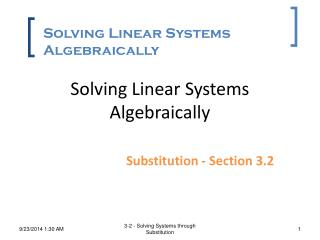DownloadDownload PresentationSolving Linear Systems Algebraically

# Solving Linear Systems Algebraically

Download Presentation## Solving Linear Systems Algebraically

- - - - - - - - - - - - - - - - - - - - - - - - - - - E N D - - - - - - - - - - - - - - - - - - - - - - - - - - -
##### Presentation Transcript

1. Solving Linear Systems Algebraically Solving Linear Systems Algebraically Substitution - Section 3.2 3-2 - Solving Systems through Substitution

2. Steps in Substitution • Steps: • SOLVE for one equation into one variable • REPLACE one equation into other equation • SUBSTITUTE the value into either equation • CHECK the solution 3-2 - Solving Systems through Substitution

3. Example 1 • Solve using Substitution • 1. SOLVE for one equation into one variable 3-2 - Solving Systems through Substitution

4. 2. REPLACE one equation into other equation 3-2 - Solving Systems through Substitution

5. 3. SUBSTITUTE the value into either equation 3-2 - Solving Systems through Substitution

6. Example 1 • Solve using Substitution 4. CHECK the solution 3-2 - Solving Systems through Substitution

7. Example 2 • Solve using Substitution 3-2 - Solving Systems through Substitution

8. Example 2 • Solve using Substitution 4. CHECK the solution 3-2 - Solving Systems through Substitution

9. Your Turn • Solve using Substitution 3-2 - Solving Systems through Substitution

10. Example 3 • Solve using Substitution 3-2 - Solving Systems through Substitution

11. Example 4 A coffee blend contains Sumatra beans which cost \$5/lb, and Kona beans, which cost \$13/lb. If the blend costs \$10/lb, how much of each type of coffee is in 50 lb of the blend? Let x represent the amount of the Sumatra beans in the blend. Let y represent the amount of the Kona beans in the blend. 3-2 - Solving Systems through Substitution

12. Amount of Sumatra beans amount of Kona beans equals 50. plus = + x y 50 Cost of Sumatra beans cost of Kona beans cost of beans. equals plus + = 13y 10(50) 5x Example 4 A coffee blend contains Sumatra beans which cost \$5/lb, and Kona beans, which cost \$13/lb. If the blend costs \$10/lb, how much of each type of coffee is in 50 lb of the blend? Write one equation based on the amount of each bean: Write another equation based on cost of the beans: 3-2 - Solving Systems through Substitution

13. x + y = 50 5x + 13y = 500 Example 4 A coffee blend contains Sumatra beans which cost \$5/lb, and Kona beans, which cost \$13/lb. If the blend costs \$10/lb, how much of each type of coffee is in 50 lb of the blend? Solve the system. First equation x + y = 50 y = 50 – x Solve the first equation for y. 5x + 13(50 – x) = 500 Substitute (50 – x) for y. 5x + 650 – 13x = 500 Distribute. –8x = –150 Simplify. x = 18.75 3-2 - Solving Systems through Substitution

14. x + y = 50 5x + 13y = 500 Example 4 A coffee blend contains Sumatra beans which cost \$5/lb, and Kona beans, which cost \$13/lb. If the blend costs \$10/lb, how much of each type of coffee is in 50 lb of the blend? Solve the system. Substitute x into one of the original equations to solve for y. Substitute the value of x into one equation. 18.75 + y = 50 y = 31.25 y = 31.25 Solve for y. The mixture will contain 18.75 lb of the Sumatra beans and 31.25 lb of the Kona beans. (18.75, 31.25) 3-2 - Solving Systems through Substitution

15. Assignment Page 194 (2-5, 15-18) 3-2 - Solving Systems through Substitution